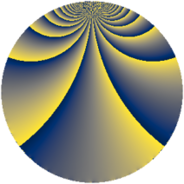# Properties

 Label 165.2.rLevel $165$ Weight $2$ Character orbit 165.r Rep. character $\chi_{165}(29,\cdot)$ Character field $\Q(\zeta_{10})$ Dimension $80$ Newform subspaces $1$ Sturm bound $48$ Trace bound $0$

# Related objects

## Defining parameters

 Level: $$N$$ $$=$$ $$165 = 3 \cdot 5 \cdot 11$$ Weight: $$k$$ $$=$$ $$2$$ Character orbit: $$[\chi]$$ $$=$$ 165.r (of order $$10$$ and degree $$4$$) Character conductor: $$\operatorname{cond}(\chi)$$ $$=$$ $$165$$ Character field: $$\Q(\zeta_{10})$$ Newform subspaces: $$1$$ Sturm bound: $$48$$ Trace bound: $$0$$

## Dimensions

The following table gives the dimensions of various subspaces of $$M_{2}(165, [\chi])$$.

Total New Old
Modular forms 112 112 0
Cusp forms 80 80 0
Eisenstein series 32 32 0

## Trace form

 $$80 q + 4 q^{4} - 10 q^{6} - 10 q^{9} + O(q^{10})$$ $$80 q + 4 q^{4} - 10 q^{6} - 10 q^{9} - 2 q^{15} - 60 q^{16} - 20 q^{19} - 30 q^{24} + 6 q^{25} + 10 q^{30} - 20 q^{31} - 56 q^{34} + 2 q^{36} - 50 q^{39} + 60 q^{40} - 92 q^{45} - 40 q^{46} + 72 q^{49} + 30 q^{51} + 4 q^{55} + 54 q^{60} + 96 q^{64} - 42 q^{66} + 30 q^{69} - 86 q^{70} + 2 q^{75} - 66 q^{81} + 140 q^{84} - 30 q^{85} + 120 q^{90} + 48 q^{91} + 60 q^{94} - 70 q^{96} + 60 q^{99} + O(q^{100})$$

## Decomposition of $$S_{2}^{\mathrm{new}}(165, [\chi])$$ into newform subspaces

Label Dim. $$A$$ Field CM Traces $q$-expansion
$$a_2$$ $$a_3$$ $$a_5$$ $$a_7$$
165.2.r.a $$80$$ $$1.318$$ None $$0$$ $$0$$ $$0$$ $$0$$